# Approaches for Calibrating Agent-Based Models to Data

### Daniel Faissol (16-FS-041)

#### Abstract

Model calibration is the process of estimating the input parameters of a simulation model to best fit an existing data set according to pre-specified criteria (for example, an objective or loss function). Model calibration, an essential step in the broader simulation model development process, is often a bottleneck to progress in model development. Current model calibration practices range from manually tuning parameters, to brute-force search methods, to more sophisticated optimization algorithms. An underlying challenge in model calibration is the “curse of dimensionality,” which characterizes problems in which the search space (and therefore computation time) increases drastically—often exponentially—with the number of parameters being simultaneously estimated. Another complication is the prevalence of high uncertainty in the face of stochastic simulations, a limitation which Bayesian approaches address by explicitly representing uncertainty in estimated values. Model calibration is particularly challenging in the biological domain, in which systems are complex (that is, dimensionality is high), interactions are inherently stochastic, and uncertainty in measurements is high. Leveraging the high-performance parallel computing resources at Lawrence Livermore National Laboratory, we evaluated the effectiveness of two optimization algorithms in performing model calibration using agent-based models of complex biological systems. Agent-based models are an especially challenging yet ideally suitable platform for exploring model calibration methods, due to their ability to represent stochastic interactions, high-dimensional spatial heterogeneity, and complex dynamics.

#### Background and Research Objectives

We sought to determine the feasibility of using two existing techniques individually for parameter estimation of agent-based models (ABMs) given a fixed set of agent rules and synthetic data. The first technique we tried was an approximate Bayesian computation (ABC) rejection algorithm. ABCs are a class of methods for performing Bayesian inference when the likelihood function is unknown, intractable, or computationally expensive. This regime is especially relevant for stochastic models. The second technique was a gradient estimation with standard simultaneous perturbations. Because the standard practice is to manually and qualitatively calibrate ABMs to data, this study can have broad implications for ABM users and potentially lead to the use of such algorithms across several communities that use ABMs. Our results demonstrated that the ABC-rejection algorithm successfully performs parameter estimation for agent-based models on a massively parallelized scale (based on the metrics described below). We also determined that gradient estimation with standard simultaneous perturbations performs poorly for model calibration for the ABM used in our study, and, likely, for similar ABMs. However, the lessons we learned allowed us to develop a better performing approach based on a central approximation and checking many step sizes within each iteration.

##### Description of the agent-based model

Our primary testbed is an agent-based model (ABM) of the innate immune response and the development of possible treatments for sepsis (An 2004). A long-term goal of the model is to predict patient outcome given a series of clinical interventions. Parameter inference is crucial to this end goal as it facilitates the connection between the ABM and real, clinical data.

The simulation structure consists of a 101×101 grid. An endothelial cell (EC) agent resides on each grid point. (Endothelial cells line the inside of every blood vessel in the body.) Each EC has a state variable oxy ∈ [0, 100] that represents its “health” status and a state variable infection ∈ [0, 100] that represents its degree of infection. At the start of the simulation, an initial injury is imposed and injured ECs have their infection set to 100. All other ECs begin with infection = 0. All ECs begin with oxy = 100.

At each time step, agents execute their rules (outlined in detail in An 2004). Notably, an EC’s behavior depends on its oxy value: oxy = 60 (healthy); 30 < oxy < 60 (ischemic or deficient in blood supply); oxy = 30 (infarcted or deprived of its blood supply). There are several other agent types including polymorphonuclear granulocytes (a category of white blood cells), monocytes (another type of white blood cell), and various T cells (lymphocytes that actively participating in the immune response), which heal ECs and regulate a series of cytokine mediators, which help generate immune responses. After 100 time steps, antibody is applied, which multiplies the infection of all ECs by the input parameter antibioticMultiplier. Each time step maps to roughly 7 minutes of real time. The simulation runs for 515 time steps (~60 hours of real time).

There are many simulation input parameters. For inferences here, we focus on the following four:

• oxyHeal ∈ [0, 100], the amount by which non-ischemic cells increase their oxy value each time step
• antibioticMultiplier ∈ [0, 1], the factor by which infection of all ECs changes when antibiotic is applied (after the first 100 time steps)
• injNumber ∈ [0..72], the size of the initial infectious injury
• infectSpread ∈ [0, 100], the amount by which an infected EC increases infection of its neighboring ECs each time step.

At the end of each time step t, the simulation outputs 16 variables:

• Variable 1: oxyDeficit(t) = ∑cells [oxy(0) − oxy(t)], a measure of systemic loss in health
• Variable 2: total Infection(t) = ∑cells infection(t), a measure of systemic infection
• Variables 3–16: cj (t) = Σcells j(t), a measure of systemic levels of cytokine j (there are 14 different cytokines)
##### Approximate Bayesian computation rejection algorithm

Rather than directly computing the likelihood function, ABC methods approximate it using a simulation model. By comparing simulation results with real data, one can perform an approximated Bayesian parameter inference. Perhaps the simplest ABC method is the ABC rejection (ABCR) algorithm. In ABCR, a simulation model is repeatedly executed using parameters independently sampled from prior distributions. The distance between the real data D and the simulated data is calculated using some specified distance function, and the sample is then only accepted if this distance falls below some threshold level, ε; otherwise it is rejected (discarded). That is, a sample is accepted if: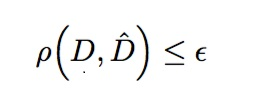This process is repeated many times; the accepted parameter samples then form an approximation of the posterior distributions. Because samples are independent, ABCR is highly parallelizable and suitable for high-performance computing. Often, calculating the “full” distance between real and simulated data is prohibitively expensive and/or memory-intensive. Thus, most ABCR approaches instead compare summary statistics, S(·), of the real and simulated data. In this case, a sample is accepted if: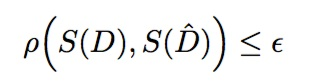For stringent threshold requirements, the acceptance rate of samples may be extremely low. An alternative approach is to specify a fixed acceptance rate, alpha (α), then accept only the best-performing fraction alpha of all samples. In this case, samples cannot be accepted or rejected until all samples have been taken.

While simple in concept, the ABCR algorithm itself has a large number of choices and parameters: (1) the choices of rho (ρ) and epsilon (ε) directly affect which samples will be accepted; (2) the choice of prior distributions, if not selected carefully, can bias the posteriors; (3) the prior distributions themselves must be parameterized; (4) the choice of summary statistics introduces bias, and they must be chosen carefully to minimize the loss of information; (5) for stochastic simulation models, the number of Monte Carlo (MC) trials per sample is a balance between uncertainty and computational cost. We applied ABCR to two simple agent-based model (ABM) testbeds, and then more thoroughly to an existing ABM of bacterial sepsis.

As a proof of concept, instead of using “real” (i.e. wet-lab or clinical) data as the basis of inference, we instead use synthetic (simulated) data that we treat as “ground truth.” The advantage of this approach is that we know the true parameter values, and thus can better assess what constitutes successful inference. Specifically, we compute the highest posterior density (HPD) regions and the Kullback-Liebler divergence. HPD regions are a subset of the parameter space for which posterior density is highest. Specifically, the 100 ∈ (1 − α)% HPD region is the subset of parameter space s(y) in theta such that: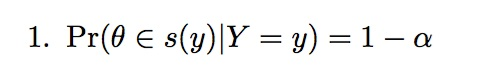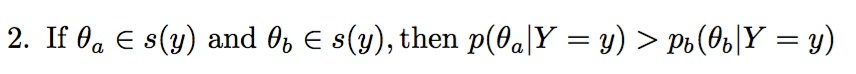The Kullback-Liebler divergence, DKL(P||Q), is a measure of the information gained by moving from the prior distribution Q to the posterior distribution P. For discretized probability distributions P and Q, the Kullback-Liebler divergence from Q to P is: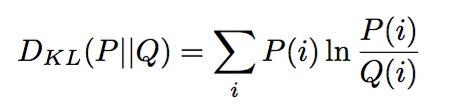#### Scientific Approach and Accomplishments

We explored two general approaches:

1. Define a small set of hand-crafted summary statistics for each output, then compare summary statistics using Equation 2.
2. Directly compare the full, time series data sets using Equation 1.

The challenge in Approach 1 is selecting summary statistics that compactly contain the relevant information in the full, time series data; comparing the summary statistics is then straightforward using a normalizing distance function like the Mahalanobis distance. For example, if a time series output looks like exponential decay, it can be fit to the curve N(t) = N0exp(t) and the parameters N0 and can be used as summary statistics.

In contrast, the challenge in Approach 2 is selecting a suitable, multivariate, time-series distance function and a method of normalizing the distance that is robust to data of different scales. For time series data, it is common to simply treat each time series output as a vector, then compute the sum over all outputs of the squared Euclidean distances between the simulated and real data; however, if outputs exist on very different scales, some outputs may dominate others. Therefore, each output can be normalized (for example, by the mean of the data) to make them scale-invariant.

In general, we found Approach 1 to be most effective in estimating a small subset of parameters, but lacking in its ability to simultaneously estimate all four parameters. For example, we defined a summary statistic as the fold-change in totalInfection following dose of antibiotic; this summary statistic alone produced an extremely sharp posterior distribution for antibioticMultiplier (DKL = 4.385). In contrast, Approach 2 was more broadly effective for all parameters but did not yield an exceptionally narrow posterior distribution for any one parameter.

To overcome the limitations of each approach, we developed a hybrid approach that takes advantage of the full time series data as in Approach 2, yet allows the by-hand customization of Approach 1. Specifically, we considered the L2 distance metric for output i as a special type of summary statistic, denoted Si, in which the MC-averaged data D acts like a parameter: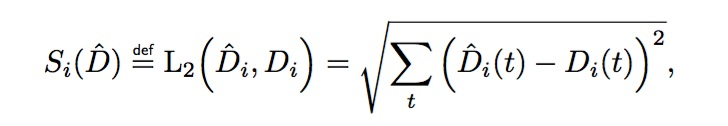where Di(t) (or D̂i(t)) is the MC-averaged real (or simulated) data of the ith output at time t.

In order to compute Equation 2 using the L2 “summary statistic,” we need to choose a distance function and calculate the corresponding summary statistic of the real data. It can be seen from the equation above that the summary statistic of the data, Si(D), is equal to zero for all outputs. Intuitively, this occurs because the information gained from the real data is already embedded into Si(D̂) because this “summary statistic” was parameterized by the real data.

For distance function rho, we chose the standardized Euclidean distance, in which each squared deviation is normalized by the variance across individual MC trials of the synthetic data. That is,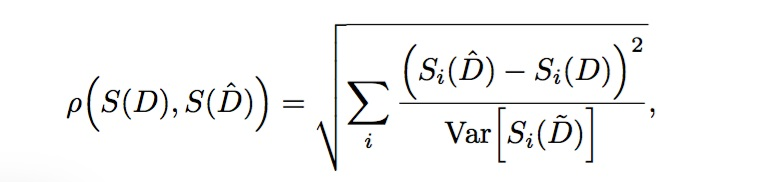where S represents a vector of summary statistics Si for each output, and D(t) is the value of an individual MC trial of the real data at time t. Note that this equation is really a distance of distances, and that the outer L2 function is applied over the various types of outputs, whereas the inner L2 functions Si apply over the time series vectors.

The advantage to this approach is two-fold. Firstly, it is both translation and scale invariant. That is, normalization techniques of the form D’ = α (I-ß ) (where α and β are constants derived from the real data) used in the inner distance function do not affect results. Translation invariance is both a property of the L2 distance metric and variance. Scale invariance occurs because both the L2 summary statistic and the variance scale such that the constant α cancels in the normalization: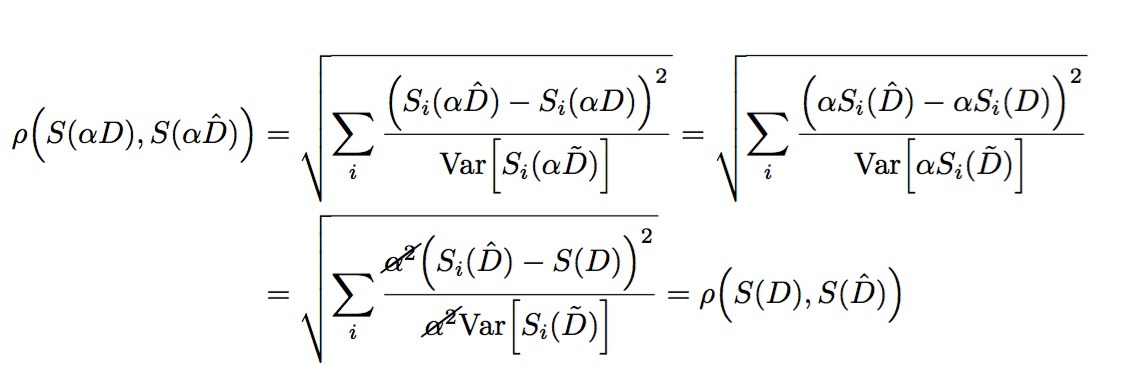Secondly, the approach allows additional, hand-crafted summary statistics to be included in the final distance metric. Specifically, in addition to the L2 summary statistics for each output i, this equation can be summed over additional summary statistics (which may be scaled differently). Note that translation and scale invariance will only hold if the additional summary statistics also exhibit translation and scale invariance as in the proof above.

##### Parameter inference for the sepsis model

We established uninformed priors for antibioticMultiplier: U[0, 1); injNumber: Ud[0,72]; and infectSpread: U[0,100). For oxyHeal, we restricted the maximum value to 1.0: U[0,1). For the synthetic data, we averaged 1,000 MC trials using the parameter values antibioticMultiplier = 0.384, injNumber = 37, infectSpread = 12.0, and oxyHeal = 0.2. These parameters were selected as a sample case that represents early phases of systemic inflammatory response syndrome (SIRS)/multiple organ failure (MOF), in which the infection completely clears but system health recovers only very slowly.

We executed 300,000 samples (each a single MC trial) from the prior distribution across 10 Aztec nodes, each using 16 cores. Each simulation took around 7 seconds per core. We first tested the limits of using the full, time series data sets to determine distance. Posterior distributions using L2 “summary statistics” for each output and the standardized Euclidean distance metric are shown in Figure 1. All four parameters are predicted reasonably well, with the modes of both marginal and pairwise joint posterior distributions falling very close to the true values. As a metric of how well we could infer the parameters, we sought the smallest HPD region which still captured the true value. Specifically, the 35% HPD regions of the marginal posterior distributions capture the true value for all parameters. Moreover, the 20% HPD regions of the pairwise joint posterior distributions capture the true value for all pairwise parameter combinations.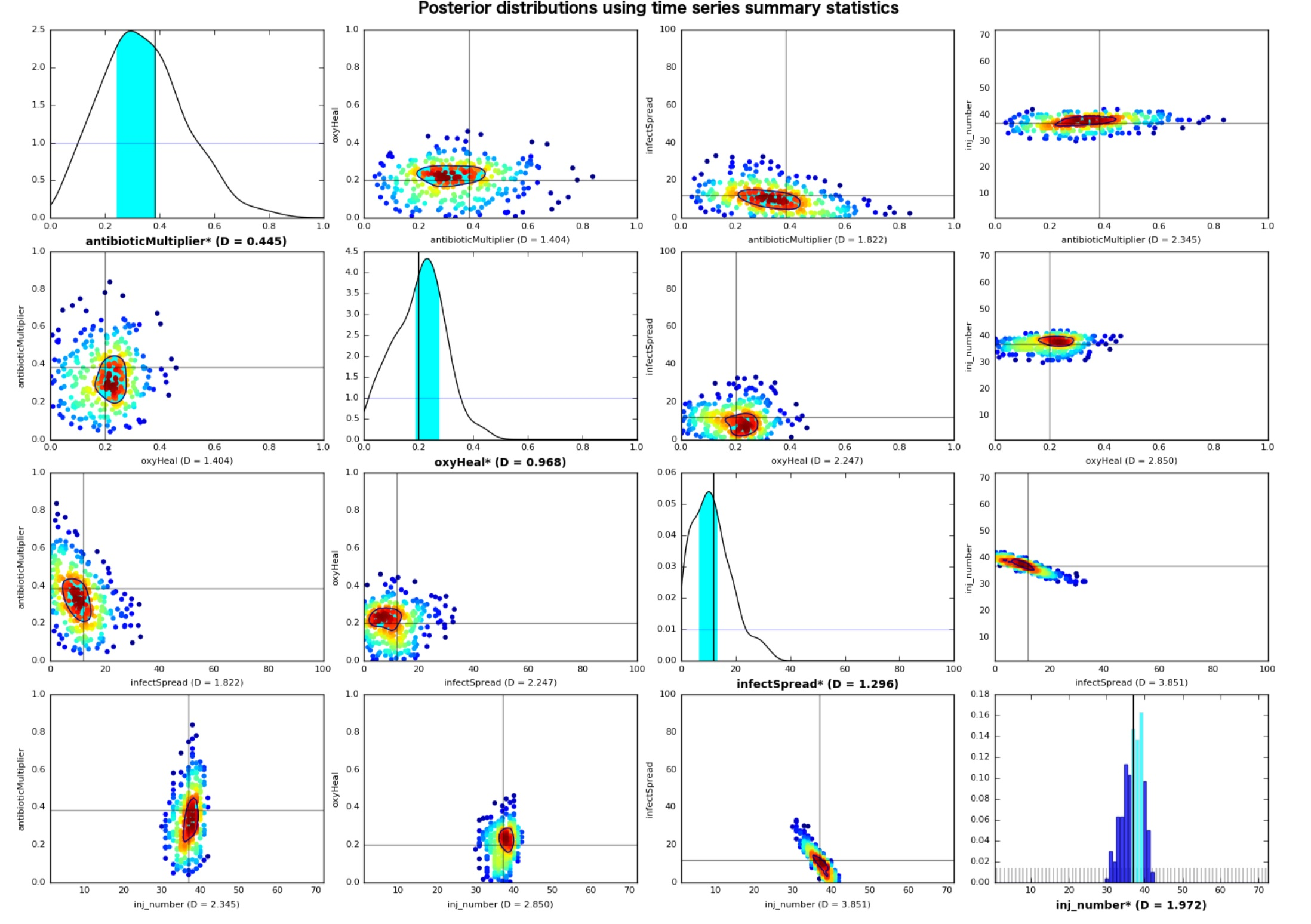Posterior distributions using L2 “summary statistics” for each output and the standardized Euclidean distance metric. Along the diagonal are marginal posterior distributions for each parameter. Prior distributions are shown as blue lines (continuous) or gray bars (discrete). For continuous parameters, marginal posterior distributions were smoothed using kernel-density estimation. Black vertical lines represent the true value. Off-diagonal plots are joint posterior distributions for pairwise combinations of parameters. Individual accepted samples are shown as points, colored according to a two-dimensional kernel density estimation. Black vertical and horizontal lines represent the true values. For all plots, the highest posterior density (HPD) regions (35% HPD intervals for marginal distributions; 20% HPD contours for pairwise joint distributions) are filled in aqua and capture the true value. An asterisk following the parameter name signifies that the true value falls within the HPD region. The Kullback-Liebler divergence (DKL) is listed for each distribution. Samples with distance in the 0.1%-quantile were accepted (N = 300,000).

We attempted other combinations; for example, including the “fold-change” summary statistic described earlier along with the L2 summary statistics produced a much sharper marginal posterior distribution for antibioticMultiplier (DKL = 2.963, compared to 0.445 without); however, the remaining three marginal posterior distributions became shallower. Similar tradeoffs were found using different combinations of summary statistics; the results in Figure 1 are a representative case of one of the top-performing combinations.

One avenue we are currently exploring is to logically AND different sets of summary statistics together when choosing whether to accept samples. That is,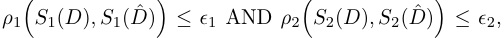is a sample is accepted if where S1 and S2 are different sets of summary statistics, potentially compared using different distance functions and thresholds. While this approach should partially avoid the tradeoffs mentioned above, a potential pitfall is that the AND operation can severely reduce the acceptance rate, thereby increasing computational cost.

Finally, we note that our efforts in developing a generalized distance function—which incorporates the full time series data as well as hand-crafted summary statistics, wrapped in a standardized Euclidean distance metric—can be used directly as the objective function for most other optimization techniques.

#### Simultaneous perturbation stochastic approximation

We also analyzed the effectiveness of simultaneous perturbation stochastic approximation (SPSA) in estimating parameters of the sepsis ABM (Spall 2003). We considered two input parameters (oxyHeal and antibioticMultiplier) and constructed the objective function from two output variables (oxyDeficit and totalInfection). Both input parameters and both output variables are continuous. The objective function was the L2 norm between the known (synthetic) and optimized output values at the end of the simulation (after 515 time steps).

The domain of antibioticMultiplier is [0,1]. The domain of oxyHeal is [0,100]; however, we incorporated prior knowledge by restricting this search space to [0,1]. For all tests, the initial point was the center of the domain, i.e. (0.5, 0.5). Each simulation output is an average of 100 Monte Carlo trials. The tolerance used as the criterion for convergence was 50, which was empirically verified as a valid upper bound on the objective function for different evaluations of the same point. We ran the SPSA algorithm on 10 different parameter sets, using Bernoulli distributions to generate the perturbation factor. We tried several step size sequences. SPSA did not converge for any parameter sets.

We also ran a gradient method using the central approximation scheme for several step size sequences, yet still did not achieve convergence for any parameter sets. Convergence was not achieved for sequences of fixed step sizes because of the high sensitivity of the objective function for some points in the domain. Since both SPSA and pure gradient methods did not work with these continuous parameters, we did not attempt to apply them to the integer parameters.

We modified the gradient method by allowing it to alter step sizes (inexact line search) and try different directions before each update. For each iteration, we tried at most 10 different directions and, for each direction, at most 10 different step sizes, until a point for which the objective function decreases was found. This procedure yielded convergence for all 10 parameter sets using the tolerance of 50.

Table 1 contains the results for each parameter set. For most parameter sets, the optimized value for oxyHeal was approximated very close to the true value, whereas antibioticMultiplier was only close for four parameter sets. This discrepancy may be caused by the choice of tolerance, the existence of multiple optima, or an underlying identifiability issue since the objective function takes into account only a subset of the simulation’s output variables.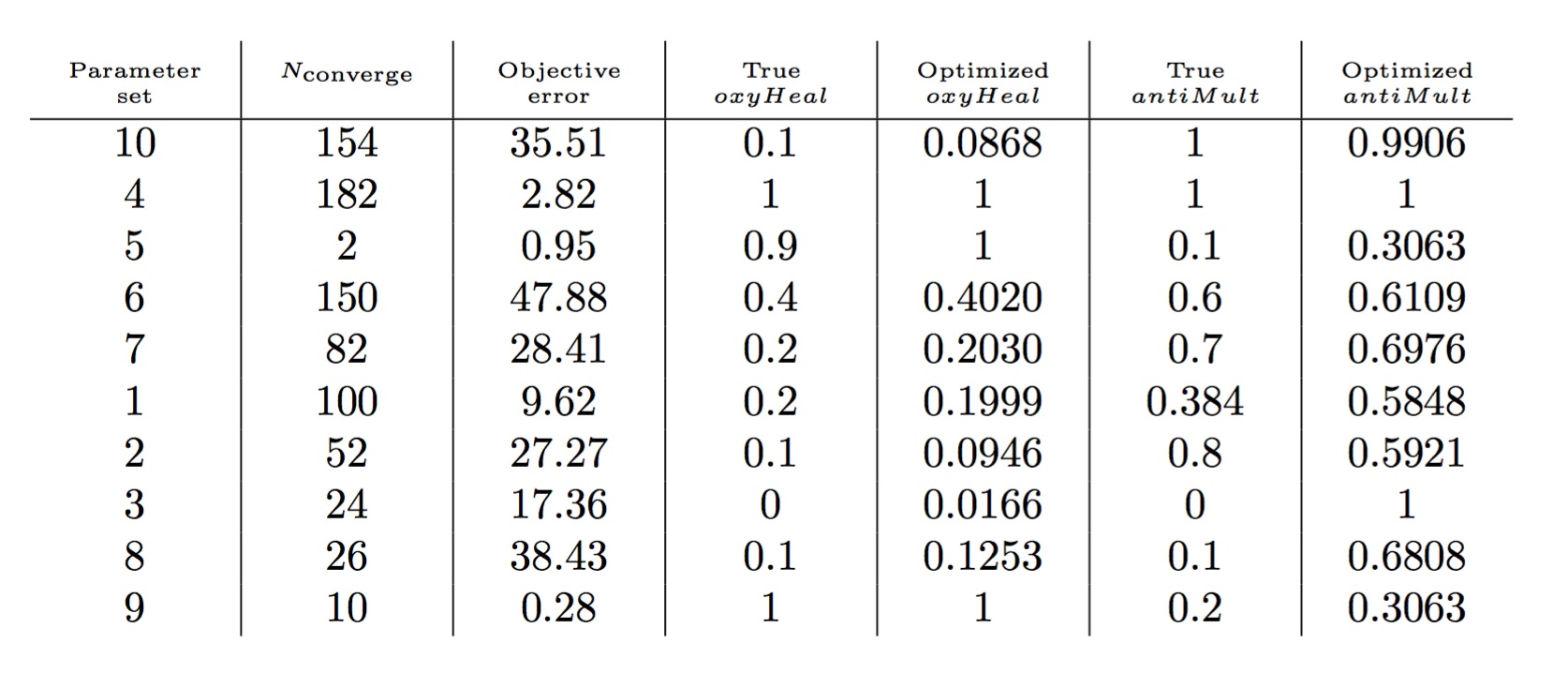Table 1. Parameter set results showing optimized values for input parameters oxyHeal and antibioticMultiplier.

#### Impact on Mission

This work addresses the NNSA goal of strengthening the science, technology, and engineering base by expanding and applying our science and technology capabilities to deal with broader national security issues. Our project also directly supports Livermore’s biosecurity mission area in that it is aimed at enabling the calibration of stochastic models in biology that would allow us to better develop countermeasures against various threats such as emerging pathogens and synthetic biological agents. The work is also highly relevant to agent-based modeling efforts in other national security related applications such as mobile sensors, cyber networks, vehicle traffic, crowd behavior, economic systems and financial markets.

#### Conclusion

We concluded that stochastic optimization techniques are not only feasible but also promising for model calibration of agent-based models of biological systems. In particular, ABCR works well in accurately predicting parameters based on multidimensional time series data even with uninformed priors. While “out-of-the-box” SPSA did not perform well, variations of other gradient-based techniques may improve results. We hypothesize that additional Bayesian and/or gradient-free methods (for example, ABC Markov chain Monte Carlo; Gaussian process modeling) will provide the best estimates of unknown parameters in the face of considerable uncertainty.

#### References

An, G. 2004. "In Silico Experiments of Existing and Hypothetical Cytokine-Directed Clinical Trials Using Agent-Based Modeling." Critical Care Medicine 32 (10):2050–2060.

Spall, J. C. "Simultaneous Perturbation Stochastic Approximation." Introduction to Stochastic Search and Optimization: Estimation, Simulation, and Control. 176–207. Hoboken: John Wiley & Sons, 2003.